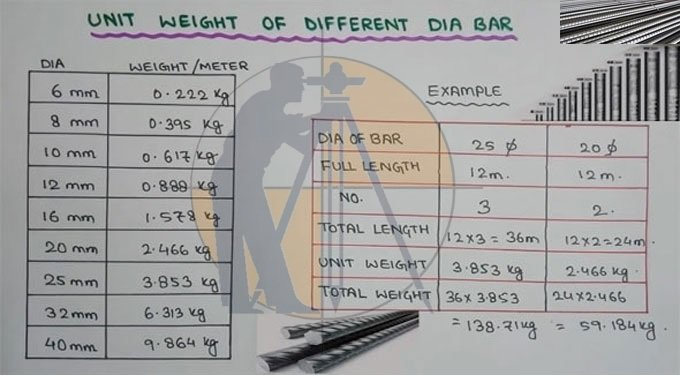Breaking News
Home / Civil Engineering / Work Out The Unit Weight Of Steel

# Work Out The Unit Weight Of Steel

## How To Work Out The Unit Weight Of Steel

Reinforcement bars are measured, obtained and paid by unit of weight i.e. in kilograms, pounds, tonnes etc. Unit weight of deformed rebars denotes its weight per unit length. The common units applied for recording unit weight of steel range from kilogram per meter (kg/m) and pounds per feet (lb/ft).

In any construction project, if there are reinforcing bars, the contractor provides the bar bending schedules to measure the quantity of rebar where details are given for the cut length of bar, diameter and weight of the rebar.

The site engineer examines and approves these bar bending schedules for addition in IPC of the Contractor which is then disbursed by the Employer.

Steel Weight Calculation Formula – The weight of steel is calculated with the following formula:Unit weight of steel = d*d/162.28 (kg/m)

This formula gives rough estimation of the unit weight of rebar, but it can’t provide you the proper value of the steel unit weight.

To get the exact result, it is required to follow the steel manufacturer’s manual where the unit weight of the steel bars is cited.

Derivation of Rebar Unit Weight Formula D2/162.28

Unit weight of steel = D2 / 162.28

This formula is originated from the density of the steel rebar that is 7850 Kg/m3.

D stands for the diameter of the rebar in mm.

The result of this formula will come as weight per length i.e. kg per meter.

By multiplying the density (ρ) with the cross-section area of the rebar, the weight per length of rebar will be produced.

Therefore;
Ρ x A = (7850 kg/m3 ) x (π x D2)/4
= (7850 kg/m3 ) x (3.14 x D2)/4
=6162.25 x D2 kg/m (where D is in meters)

As φ diameter of rebar is commonly provided in millimeters.

So, let’s assume Dm is diameter in mm and to change it into meters, it should be divided with 1000.

Thus;
1 meter = 1000 millimeters
=6162.25 x (Dm/1000)2 kg/m
=6162.25 x (Dm/1000)2 kg/m
=0.00616225 x Dm2

Or
= Dm2/(0.00616225)-1
=Dm2/162.2783 in kg/m

Where Dm stands for dia of rebar in mm.

Therefore, this formula is applicable for getting fairly accurate value of unit weight of steel in MKS system.

### THANKS.